List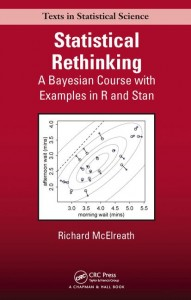Here I work through the practice questions in Chapter 2, “Small Worlds and Large Worlds,” of Statistical Rethinking (McElreath, 2016). I do my best to use only approaches and functions discussed so far in the book, as well as to name objects consistently with how the book does. If you find any typos or mistakes in my answers, or if you have any relevant questions, please feel free to add a comment below.

Here is the chapter summary from page 45:

This chapter introduced the conceptual mechanics of Bayesian data analysis. The target of inference in Bayesian inference is a posterior probability distribution. Posterior probabilities state the relative numbers of ways each conjectured cause of the data could have produced the data. These relative numbers indicate plausibilities of the different conjectures. These plausibilities are updated in light of observations, a process known as Bayesian updating.

More mechanically, a Bayesian model is a composite of a likelihood, a choice of parameters, and a prior. The likelihood provides the plausibility of each possible value of the parameters, before accounting for the data. The rules of probability tell us that the logical way to compute the plausibilities, after accounting for the data, is to use Bayes’ theorem. This results in the posterior distribution.

In practice, Bayesian models are fit to data using numerical techniques, like grid approximation, quadratic approximation, and Markov chain Monte Carlo. Each method imposes different trade-offs.

# Setup
library(rethinking)
set.seed(2)


## Easy Difficulty

### Practice Question 2E1

Which of the expressions below correspond to the statement: the probability of rain on Monday?

1. $$\Pr(\mathrm{rain})$$
2. $$\Pr(\mathrm{rain} | \mathrm{Monday})$$
3. $$\Pr(\mathrm{Monday} | \mathrm{rain})$$
4. $$\Pr(\mathrm{rain}, \mathrm{Monday}) / \Pr(\mathrm{Monday})$$

First, let’s interpret each expression:

Option 1 is the probability of rain.

Option 2 is the probability of rain, given that it is Monday.

Option 3 is the probability of it being Monday, given rain.

Option 4 is the probability of rain and it being Monday, given that it is Monday.

The correct answers are Option 2 and Option 4 (they are equal).

This equivalence can be derived using algebra and the joint probability definition on page 36:
$\Pr(w,p)=\Pr(w|p)\Pr(p)$
Although it will be easier to see if we rename $$w$$ to $$\mathrm{rain}$$ and $$p$$ to $$\mathrm{Monday}$$:
$\Pr(\mathrm{rain},\mathrm{Monday})=\Pr(\mathrm{rain}|\mathrm{Monday})\Pr(\mathrm{Monday})$

Now we divide each side by $$\Pr(p)$$ to isolate $$\Pr(\mathrm{rain}|\mathrm{Monday})$$:
$\frac{\Pr(\mathrm{rain},\mathrm{Monday})}{\Pr(\mathrm{Monday})} = \frac{\Pr(\mathrm{rain}|\mathrm{Monday})\Pr(\mathrm{Monday})}{\Pr(\mathrm{Monday})}$
The $$\Pr(\mathrm{Monday})$$ in the numerator and denominator of the right-hand side cancel out:
$\frac{\Pr(\mathrm{rain},\mathrm{Monday})}{\Pr(\mathrm{Monday})} = \Pr(\mathrm{rain}|\mathrm{Monday})$

### Practice Question 2E2

Which of the following statements corresponds to the expression: $$\Pr(\mathrm{Monday} | \mathrm{rain})$$?

1. The probability of rain on Monday.
2. The probability of rain, given that it is Monday.
3. The probability that it is Monday, given that it is raining.
4. The probability that it is Monday and that it is raining.

Let’s convert each statement to an expression:

Option 1 would be $$\Pr(\mathrm{rain} | \mathrm{Monday})$$.

Option 2 would be $$\Pr(\mathrm{rain} | \mathrm{Monday})$$.

Option 3 would be $$\Pr(\mathrm{Monday} | \mathrm{rain})$$.

Option 4 would be $$\Pr(\mathrm{Monday}, \mathrm{rain})$$.

The correct answer is Option 3 only.

Using the approach from 2E1, we could show that Option 4 is equal to $$\Pr(\mathrm{Monday}|\mathrm{rain})\Pr(\mathrm{rain})$$, but that is not what we want.

### Practice Question 2E3

Which of the expressions below correspond to the statement: the probability that it is Monday, given that it is raining?

1. $$\Pr(\mathrm{Monday}|\mathrm{rain})$$
2. $$\Pr(\mathrm{rain}|\mathrm{Monday})$$
3. $$\Pr(\mathrm{rain}|\mathrm{Monday})\Pr(\mathrm{Monday})$$
4. $$\Pr(\mathrm{rain}|\mathrm{Monday})\Pr(\mathrm{Monday})/\Pr(\mathrm{rain})$$
5. $$\Pr(\mathrm{Monday}|\mathrm{rain})\Pr(\mathrm{rain})/\Pr(\mathrm{Monday})$$

Let’s convert each expression into a statement:

Option 1 would be the probability that it is Monday, given that it is raining.

Option 2 would be the probability of rain, given that it is Monday.

Option 3 needs to be converted using the formula on page 36:
$\Pr(\mathrm{rain}|\mathrm{Monday})\Pr(\mathrm{Monday}) = \Pr(\mathrm{rain}, \mathrm{Monday})$
This is much easier to interpret as the probability that it is raining and that it is Monday.

Option 4 is the same as the previous option but with division added:
$\Pr(\mathrm{rain}|\mathrm{Monday})\Pr(\mathrm{Monday})/\Pr(\mathrm{rain})=\Pr(\mathrm{rain}, \mathrm{Monday})/\Pr(\mathrm{rain})$
We can now use algebra and the joint probability formula (page 36) to simplify this:
$\Pr(\mathrm{rain}, \mathrm{Monday})/\Pr(\mathrm{rain})=\Pr(\mathrm{Monday}|\mathrm{rain})$
This is much easier to interpret as the probability that it is Monday, given that it is raining.

Option 5 is the same as the previous option but with the terms exchanged. So it can be interpreted (repeating all the previous work) as the probability of rain, given that it is Monday.

The correct answers are thus Option 1 and Option 4.

### Practice Question 2E4

The Bayesian statistician Bruno de Finetti (1906-1985) began his book on probability theory with the declaration: “PROBABILITY DOES NOT EXIST.” The capitals appeared in the original, so I imagine de Finetti wanted us to shout the statement. What he meant is that probability is a device for describing uncertainty from the perspective of an observer with limited knowledge; it has no objective reality. Discuss the globe tossing example from the chapter, in light of this statement. What does it mean to say “the probability of water is 0.7”?

From the Bayesian perspective, there is one true value of a parameter at any given time and thus there is no uncertainty and no probability in “objective reality.” It is only from the perspective of an observer with limited knowledge of this true value that uncertainty exists and that probability is a useful device. So the statement, “the probability of water is 0.7” means that, given our limited knowledge, our estimate of this parameter’s value is 0.7 (but it has some single true value independent of our uncertainty).

## Medium Difficulty

### Practice Question 2M1

Recall the globe tossing model from the chapter. Compute and plot the grid approximate posterior distribution for each of the following sets of observations. In each case, assume a uniform prior for $$p$$.

1. $$W, W, W$$
2. $$W, W, W, L$$
3. $$L, W, W, L, W, W, W$$

Using the approach detailed on page 40, we use the dbinom() function and provide it with arguments corresponding to the number of $W$s and the number of tosses (in this case 3 and 3):

p_grid <- seq(from = 0, to = 1, length.out = 20)
prior <- rep(1, 20)
likelihood <- dbinom(3, size = 3, prob = p_grid)
unstd.posterior <- likelihood * prior
posterior <- unstd.posterior / sum(unstd.posterior)
plot(p_grid, posterior, type = "b",
xlab = "probability of water", ylab = "posterior probability")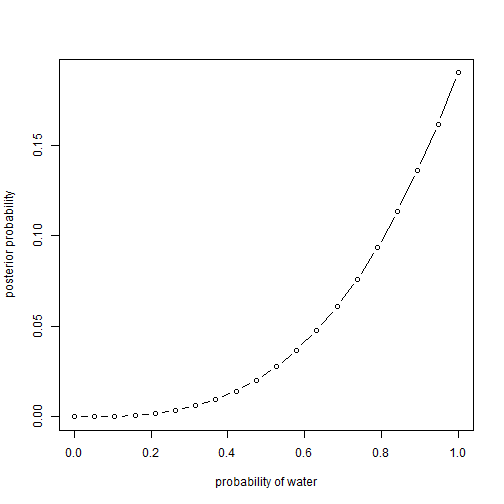We recreate this but update the arguments to 3 $W$s and 4 tosses.

p_grid <- seq(from = 0, to = 1, length.out = 20)
prior <- rep(1, 20)
likelihood <- dbinom(3, size = 4, prob = p_grid)
unstd.posterior <- likelihood * prior
posterior <- unstd.posterior / sum(unstd.posterior)
plot(p_grid, posterior, type = "b",
xlab = "probability of water", ylab = "posterior probability")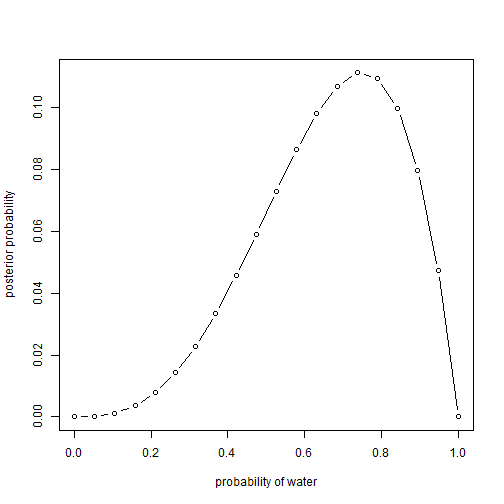Again, this time with 5 $W$s and 7 tosses:

p_grid <- seq(from = 0, to = 1, length.out = 20)
prior <- rep(1, 20)
likelihood <- dbinom(5, size = 7, prob = p_grid)
unstd.posterior <- likelihood * prior
posterior <- unstd.posterior / sum(unstd.posterior)
plot(p_grid, posterior, type = "b",
xlab = "probability of water", ylab = "posterior probability")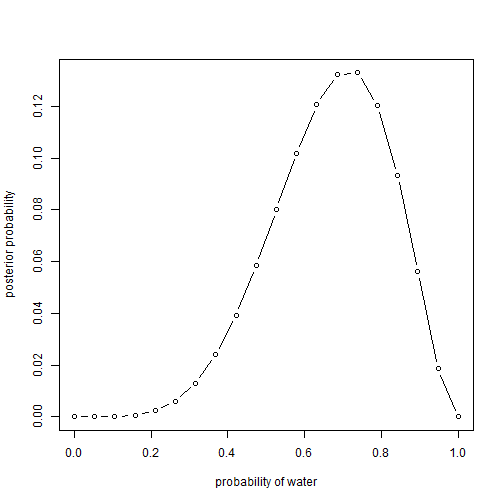### Practice Question 2M2

Now assume a prior for $$p$$ that is equal to zero when $$p<0.5$$ and is a positive constant when $$p\ge0.5$$. Again compute and plot the grid approximate posterior distribution for each of the sets of observations in the problem just above.

So we can use the same approach and code as before, but we need to update the prior. In this case, we can use the ifelse() function as detailed on page 40:

p_grid <- seq(from = 0, to = 1, length.out = 20)
prior <- ifelse(p_grid < 0.5, 0, 1)
likelihood <- dbinom(3, size = 3, prob = p_grid)
unstd.posterior <- likelihood * prior
posterior <- unstd.posterior / sum(unstd.posterior)
plot(p_grid, posterior, type = "b",
xlab = "probability of water", ylab = "posterior probability")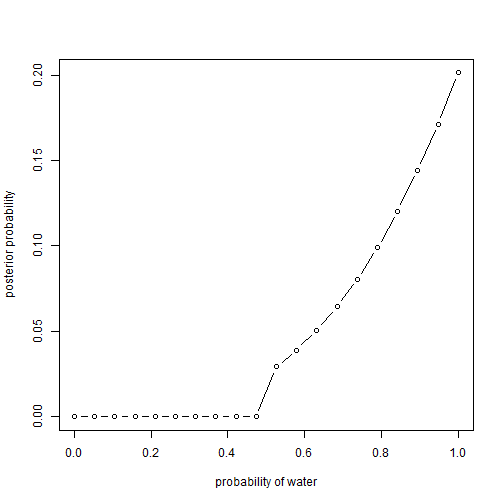p_grid <- seq(from = 0, to = 1, length.out = 20)
prior <- ifelse(p_grid < 0.5, 0, 1)
likelihood <- dbinom(3, size = 4, prob = p_grid)
unstd.posterior <- likelihood * prior
posterior <- unstd.posterior / sum(unstd.posterior)
plot(p_grid, posterior, type = "b",
xlab = "probability of water", ylab = "posterior probability")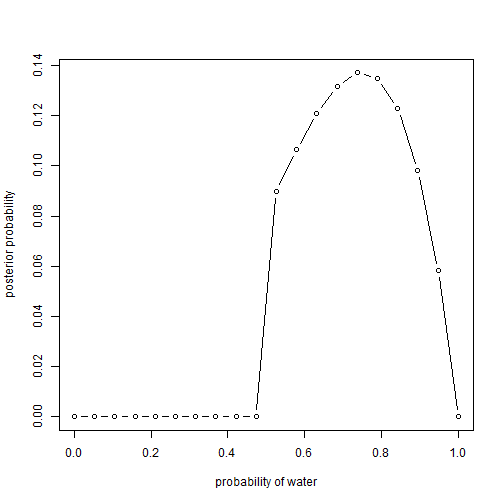p_grid <- seq(from = 0, to = 1, length.out = 20)
prior <- ifelse(p_grid < 0.5, 0, 1)
likelihood <- dbinom(5, size = 7, prob = p_grid)
unstd.posterior <- likelihood * prior
posterior <- unstd.posterior / sum(unstd.posterior)
plot(p_grid, posterior, type = "b",
xlab = "probability of water", ylab = "posterior probability")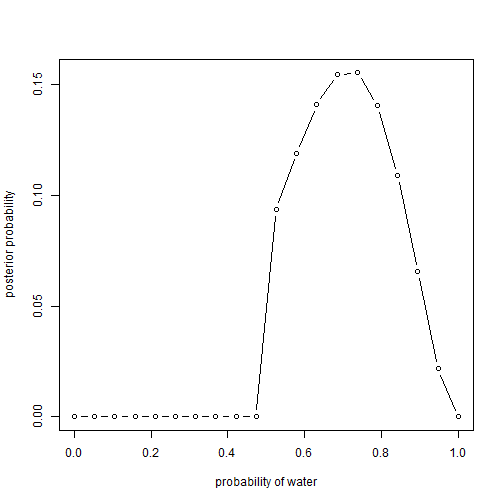Any parameter values less than 0.5 get their posterior probabilities reduced to zero through multiplication with a prior of zero. Otherwise they are the same as before.

### Practice Question 2M3

Suppose there are two globes, one for Earth and one for Mars. The Earth globe is 70% covered in water. The Mars globe is 100% land. Further suppose that one of these globes–you don’t know which–was tossed in the air and produces a “land” observation. Assume that each globe was equally likely to be tossed. Show that the posterior probability that the globe was the Earth, conditional on seeing “land” ($$\Pr(\mathrm{Earth}|\mathrm{land})$$), is 0.23.

To begin, let’s list all the information provided by the question:

$\Pr(\mathrm{land} | \mathrm{Earth}) = 1 – 0.7 = 0.3$
$\Pr(\mathrm{land} | \mathrm{Mars}) = 1$
$\Pr(\mathrm{Earth}) = \Pr(\mathrm{Mars}) = 0.5$

Now, we need to use Bayes’ theorem (first formula on page 37) to get the answer:
$\Pr(\mathrm{Earth} | \mathrm{land}) = \frac{\Pr(\mathrm{land} | \mathrm{Earth}) \Pr(\mathrm{Earth})}{\Pr(\mathrm{land})}=\frac{0.3(0.5)}{\Pr(\mathrm{land})}=\frac{0.15}{\Pr(\mathrm{land})}$

After substituting in what we know (on the right above), we still need to calculate $$\Pr(\mathrm{land})$$. We can do this using the third formula on page 37. This is called the marginal likelihood, and to calculate it, we need to take the probability of each possible globe and multiply it by the conditional probability of seeing land given that globe; we then add up every such product:
$\Pr(\mathrm{land}) = \Pr(\mathrm{land} | \mathrm{Earth}) \Pr(\mathrm{Earth}) + \Pr(\mathrm{land} | \mathrm{Mars}) \Pr(\mathrm{Mars})=0.3(0.5)+1(0.5)=0.65$
Now we can substitute this value into the formula from before to get our answer:
$\Pr(\mathrm{Earth} | \mathrm{land}) = \frac{0.15}{\Pr(\mathrm{land})}=\frac{0.15}{0.65}$

So the final answer is 0.2307692, which indeed rounds to 0.23.

### Practice Question 2M4

Suppose you have a deck with only three cards. Each card has two sides, and each side is either black or white. One card has two black sides. The second card has one black and one white side. The third card has two white sides. Now suppose all three cards are placed in a bag and shuffled. Someone reaches into the bag and pulls out a card and places it flat on a table. A black side is shown facing up, but you don’t know the color of the side facing down. Show that the probability that the other side is also black is 2/3. Use the counting method (Section 2 of the chapter) to approach this problem. This means counting up the ways that each card could produce the observed data (a black card facing up on the table).

We can represent the three cards as BB, BW, and WW to indicate their sides as being black (B) or white (W). Now we just need to count the number of ways each card could produce the observed data (a black card facing up on the table). Since BB could produce this result from either side facing up, it has two ways to produce it ($$2$$). BW could only produce this with its black side facing up ($$1$$), and WW cannot produce it in any way ($$0$$). So there are three total ways to produce the current observation ($$2+1+0=3$$). Of these three ways, only the ways produced by the BB card would allow the other side to also be black. It can be helpful to create a table:

Card Ways
BB 2
BW 1
WW 0

To get the final answer, we divide the number of ways to generate the observed data given the BB card by the total number of ways to generate the observed data (i.e., given any card):
$\Pr(\mathrm{BB})=\frac{\mathrm{BB}}{\mathrm{BB+BW+BW}}=\frac{2}{2+1+0}=\frac{2}{3}$

The probability of the other side being black is indeed 2/3.

For bonus, to do this in R, we can do the following:

card <- c("BB", "BW", "WW")
ways <- c(2, 1, 0)
p <- ways / sum(ways)
sum(p[card == "BB"])

##  0.6666667


### Practice Question 2M5

Now suppose there are four cards: BB, BW, WW, and another BB. Again suppose a card is drawn from the bag and a black side appears face up. Again calculate the probability that the other side is black.

Let’s update our table to include the new card. Like the other BB card, it has $$2$$ ways to produce the observed data.

Card Ways
BB 2
BW 1
WW 0
BB 2

We can use the same formulas as before; we just need to update the numbers:

$\Pr(\mathrm{BB})=\frac{\mathrm{BB}}{\mathrm{BB+BW+BW}}=\frac{2+2}{2+1+0+2}=\frac{4}{5}$
The probability of the other side being black is now 4/5.

Again, in R as a bonus:

card <- c("BB", "BW", "WW", "BB")
ways <- c(2, 1, 0, 2)
p <- ways / sum(ways)
sum(p[card == "BB"])

##  0.8


### Practice Question 2M6

Imagine that black ink is heavy, and so cards with black sides are heavier than cards with white sides. As a result, it’s less likely that a card with black sides is pulled from the bag. So again assume that there are three cards: BB, BW, and WW. After experimenting a number of times, you conclude that for every way to pull the BB card from the bag, there are 2 ways to pull the BW card and 3 ways to pull the WW card. Again suppose that a card is pulled and a black side appears face up. Show that the probability the other side is black is now 0.5. Use the counting method, as before.

Let’s update the table and include new columns for the prior and the likelihood. As described on pages 26-27, the likelihood for a card is the product of multiplying its ways and its prior:

Card Ways Prior Likelihood
BB 2 1 2
BW 1 2 2
WW 0 3 0

Now we can use the same formula as before, but using the likelihood instead of the raw counts.
$\Pr(\mathrm{BB})=\frac{\mathrm{BB}}{\mathrm{BB+BW+BW}}=\frac{2}{2+2+0}=\frac{2}{4}=\frac{1}{2}$
So the probability of the other side being black is indeed now 0.5.

Again, in R for bonus:

card <- c("BB", "BW", "WW")
ways <- c(2, 1, 0)
prior <- c(1, 2, 3)
likelihood <- ways * prior
p <- likelihood / sum(likelihood)
sum(p[card == "BB"])

##  0.5


### Practice Question 2M7

Assume again the original card problem, with a single card showing a black side face up. Before looking at the other side, we draw another card from the bag and lay it face up on the table. The face that is shown on the new card is white. Show that the probability that the first card, the one showing a black side, has black on its other side is now 0.75. Use the counting method, if you can. Hint: Treat this like the sequence of globe tosses, counting all the ways to see each observation, for each possible first card.

As the hint suggests, let’s fill in the table below by thinking through each possible combination of first and second cards that could produce the observed data. If the first card was the first side of BB, then there would be 3 ways for the second card to show white (i.e., the second side of BW, the first side of WW, or the second side of WW). If the first card was the second side of BB, then there would be the same 3 ways for the second card to show white. So the total ways for the first card to be BB is $$3+3=6$$. If the first card was the first side of BW, then there would be 2 ways for the second card to show white (i.e., the first side of WW or the second side of WW; it would not be possible for the white side of itself to be shown). Finally, there would be no ways for the first card to have been the second side of BW or either side of WW.

Card Ways
BB 6
BW 2
WW 0

In order for the other side of the first card to be black, the first card would have had to be BB. So we can calculate this probability by dividing the number of ways given BB by the total number of ways:
$\Pr(\mathrm{BB})=\frac{\mathrm{BB}}{\mathrm{BB+BW+WW}}=\frac{6}{6+2+0}=\frac{6}{8}=0.75$

So the probability of the first card having black on the other side is indeed 0.75.

Again, in R for bonus:

card <- c("BB", "BW", "WW")
ways <- c(6, 2, 0)
p <- ways / sum(ways)
sum(p[card == "BB"])

##  0.75


## Hard Difficulty

### Practice Question 2H1

Suppose there are two species of panda bear. Both are equally common in the wild and live in the same place. They look exactly alike and eat the same food, and there is yet no genetic assay capable of telling them apart. They differ however in family sizes. Species A gives birth to twins 10% of the time, otherwise birthing a single infant. Species B births twins 20% of the time, otherwise birthing singleton infants. Assume these numbers are known with certainty, from many years of field research.

Now suppose you are managing a captive panda breeding program. You have a new female panda of unknown species, and she has just given birth to twins. What is the probability that her next birth will also be twins?

As before, let’s begin by listing the information provided in the question:

$\Pr(\mathrm{twins} | A) = 0.1$
$\Pr(\mathrm{twins} | B) = 0.2$
$\Pr(A) = 0.5$
$\Pr(B) = 0.5$

Next, let’s calculate the marginal probability of twins on the first birth (using the formula on page 37):
$\Pr(\mathrm{twins}) = \Pr(\mathrm{twins} | A) \Pr(A) + \Pr(\mathrm{twins} | B) \Pr(B) = 0.1(0.5) + 0.2(0.5) = 0.15$

We can use the new information that the first birth was twins to update the probabilities that the female is species A or B (using Bayes’ theorem on page 37):
$\Pr(A | \mathrm{twins}) = \frac{\Pr(\mathrm{twins} | A) \Pr (A)}{\Pr(\mathrm{twins})} = \frac{0.1(0.5)}{0.15} = \frac{1}{3}$
$\Pr(B | \mathrm{twins}) = \frac{\Pr(\mathrm{twins} | B) \Pr (B)}{\Pr(\mathrm{twins})} = \frac{0.2(0.5)}{0.15} = \frac{2}{3}$

These values can be used as the new $$\Pr(A)$$ and $$\Pr(B)$$ estimates, so now we are in a position to answer the question about the second birth. We just have to calculate the updated marginal probability of twins.
$\Pr(\mathrm{twins}) = \Pr(\mathrm{twins} | A) \Pr(A) + \Pr(\mathrm{twins} | B) \Pr(B) = 0.1\bigg(\frac{1}{3}\bigg) + 0.2\bigg(\frac{2}{3}\bigg) = \frac{1}{6}$

So the probability that the female will give birth to twins, given that she has already given birth to twins is 1/6 or 0.17.

Note that this estimate is between the known rates for species A and B, but is much closer to that of species B to reflect the fact that having already given birth to twins increases the likelihood that she is species B.

### Practice Question 2H2

Recall all the facts from the problem above. Now compute the probability that the panda we have is from species A, assuming we have observed only the first birth and that it was twins.

We already computed this as part of answering the previous question through Bayesian updating.
$\Pr(A | \mathrm{twins}) = \frac{\Pr(\mathrm{twins} | A) \Pr (A)}{\Pr(\mathrm{twins})} = \frac{0.1(0.5)}{0.15} = \frac{1}{3}$

The probability that the female is from species A, given that her first birth was twins, is 1/3 or 0.33.

### Practice Question 2H3

Continuing on from the previous problem, suppose the same panda mother has a second birth and that it is not twins, but a singleton infant. Compute the posterior probability that this panda is species A.

We can use the same approach to update the probability again. To keep things readable, I will also rearrange things to be in terms of singleton births rather than twins.

$\Pr(\mathrm{single}|A) = 1 – \Pr(\mathrm{twins}|A) = 1 – 0.1 = 0.9$
$\Pr(\mathrm{single}|B) = 1 – \Pr(\mathrm{twins}|B) = 1 – 0.2 = 0.8$
$\Pr(A) = \frac{1}{3}$
$\Pr(B) = \frac{2}{3}$
$\Pr(\mathrm{single}) = \Pr(\mathrm{single}|A)\Pr(A) + \Pr(\mathrm{single}|B)\Pr(B) = 0.9(\frac{1}{3}) + 0.8(\frac{2}{3}) = \frac{5}{6}$
$\Pr(A | \mathrm{single}) = \frac{\Pr(\mathrm{single}|A)\Pr(A)}{\Pr(\mathrm{single})} = \frac{0.9(1/3)}{5/6} = 0.36$

So the posterior probability that this panda is species A is 0.36.

Note that this probability increased from 0.33 to 0.36 when it was observed that the second birth was not twins. This reflects the idea that singleton births are more likely in species A than in species B.

### Practice Question 2H4

A common boast of Bayesian statisticians is that Bayesian inferences makes it easy to use all of the data, even if the data are of different types.

So suppose now that a veterinarian comes along who has a new genetic test that she claims can identify the species of our mother panda. But the test, like all tests, is imperfect. This is the information you have about the test:

• The probability it correctly identifies a species A panda is 0.8.
• The probability it correctly identifies a species B panda is 0.65.

The vet administers the test to your panda and tells you that the test is positive for species A. First ignore your previous information from the births and compute the posterior probability that your panda is species A. Then redo your calculation, now using the birth data as well.

Using the test information only, we go back to the idea that the species are equally likely.

$\Pr(+|A) = 0.8$
$\Pr(+|B) = 0.65$
$\Pr(A) = 0.5$
$\Pr(B) = 0.5$
Now we can solve this like we have been solving the other questions:
$\Pr(+) = \Pr(+ | A) \Pr(A) + \Pr(+ | B)\Pr(B) = 0.8(0.5) + 0.65(0.5) = 0.725$
$\Pr(A | +) = \frac{\Pr(+ | A) \Pr(A)}{\Pr(+)} = \frac{0.8(0.5)}{0.725} = 0.552$

So the posterior probability of species A (using just the test result) is 0.552.

To use the previous birth information, we can update our priors of the probability of species A and B.
$\Pr(+|A) = 0.8$
$\Pr(+|B) = 0.65$
$\Pr(A) = 0.36$
$\Pr(B) = 1 – \Pr(A) = 1 – 0.36 = 0.64$

Now we just need to do the same process again using the updated values.
$\Pr(+) = \Pr(+ | A) \Pr(A) + \Pr(+ | B)\Pr(B) = 0.8(0.36) + 0.65(0.64) = 0.704$
$\Pr(A | +) = \frac{\Pr(+ | A) \Pr(A)}{\Pr(+)} = \frac{0.8(0.36)}{0.704} = 0.409$

The posterior probability of species A (using both the test result and the birth information) is 0.409.

The fact that this result is smaller suggests that the test was overestimating the likelihood of species A.

### Session Info

sessionInfo()

## R version 3.5.1 (2018-07-02)
## Platform: x86_64-w64-mingw32/x64 (64-bit)
## Running under: Windows >= 8 x64 (build 9200)
##
## Matrix products: default
##
## locale:
##  LC_COLLATE=English_United States.1252
##  LC_CTYPE=English_United States.1252
##  LC_MONETARY=English_United States.1252
##  LC_NUMERIC=C
##  LC_TIME=English_United States.1252
##
## attached base packages:
##  parallel  stats     graphics  grDevices utils     datasets  methods
##  base
##
## other attached packages:
##  ggplot2_3.1.0        knitr_1.21           RWordPress_0.2-3
##  usethis_1.4.0
##
## loaded via a namespace (and not attached):
##   tidyselect_0.2.5   xfun_0.4           purrr_0.2.5
##   lattice_0.20-35    colorspace_1.3-2   stats4_3.5.1
##   loo_2.0.0          yaml_2.2.0         XML_3.98-1.16
##  rlang_0.3.0.1      pkgbuild_1.0.2     pillar_1.3.1
##  glue_1.3.0         withr_2.1.2        bindrcpp_0.2.2
##  matrixStats_0.54.0 bindr_0.1.1        plyr_1.8.4
##  stringr_1.3.1      munsell_0.5.0      gtable_0.2.0
##  mvtnorm_1.0-8      coda_0.19-2        evaluate_0.12
##  inline_0.3.15      callr_3.1.1        ps_1.3.0
##  markdown_0.9       XMLRPC_0.3-1       highr_0.7
##  Rcpp_1.0.0         scales_1.0.0       mime_0.6
##  fs_1.2.6           gridExtra_2.3      stringi_1.2.4
##  processx_3.2.1     dplyr_0.7.8        grid_3.5.1
##  cli_1.0.1          tools_3.5.1        bitops_1.0-6
##  magrittr_1.5       lazyeval_0.2.1     RCurl_1.95-4.11
##  tibble_1.4.2       crayon_1.3.4       pkgconfig_2.0.2
##  MASS_7.3-50        prettyunits_1.0.2  assertthat_0.2.0
##  R6_2.3.0           compiler_3.5.1


### References

McElreath, R. (2016). Statistical rethinking: A Bayesian course with examples in R and Stan. New York, NY: CRC Press.

### 4 Responses to “Statistical Rethinking: Chapter 2 Practice”

1.Keh-Harng Feng

Hello.

I think the computation for 2H4 is incorrect. Specifically, if a positive test result is indication of the subject being from species A, P(+|B) should correspond to the false positive scenario where the test shows positive yet the subject is actually from species B. Thus P(+|B) = 1 – P(-|B) = 0.35.

Regards,

•iwi
•Jackson M

You’re correct, Keh-Harng.

2.Ganesh

Just in case anyone is still looking for the correct answer and has no explanation, a rewording of the statement “correctly identifies a species A panda is 0.8” helps.
The test says A, given that it is actually A is 0.8. P (test says A | A) = 0.8.
The test says B, given that it is actually B is 0.65. P(test says B | B) = 0.65.

So it becomes immediately intuitive that the probability of test saying A but it actually is B just means the probability of test being wrong about B.
P(test says A | B) = 1 – P (test says B | B) = 1 – 0.65 = 0.35

And for the posterior calculation, you would have to use
P(test says A | A) / ( P(test says A | A) + P(test says A | B) )

Keh-Harng Feng is correct.

Hope this helps!